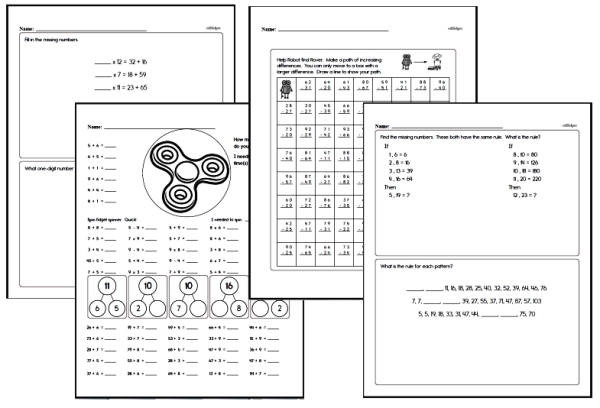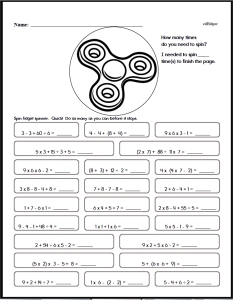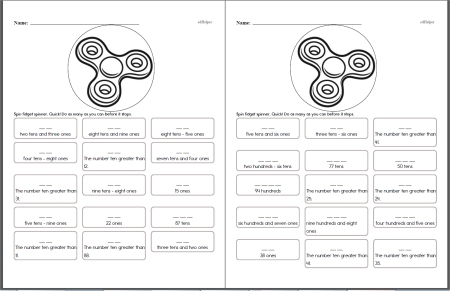# Math Facts: Free Printable PDF Worksheets

## Teacher Resources made by Other Teachers:

 Minute Math Workbooks with Fidget Spinner Math Facts PagesKindergarten Math Minutes First Grade Math Minutes Second Grade Math Minutes Third Grade Math Minutes Fourth Grade Math Minutes Fifth Grade Math Minutes Sixth Grade Math Minutes Addition Math Facts Workbook, To Sums of 18 Workbook with increasing difficulty to practice addition facts, to sums of 18 Only sum is blank Any number blank - Use subtraction and addition skills Addition Math Facts Workbook, To Sums of 99 Workbook with increasing difficulty to practice addition facts, to sums of 99 Only sum is blank Any number blank - Use subtraction and addition skills Multiplication Math Facts Workbook, To Products of 144 Workbook with increasing difficulty to practice multiplication facts Only product is blank Any number blank - Use division and multiplication skills Math Facts with Fidget Spinners Challenge Workbook Mix of everything - kids will need to use their addition, subtraction, multiplication, and division skills Order of Operations Math Facts with Fidget SpinnersOrder of Operations Worksheets - Fast Review with Fidget Spinners Place ValuePlace Value Fluency with Fidget Spinners 亚洲欧美国产综合在线一区_亚洲 欧美 日韩 国产 制服_亚洲2020天天堂在线观看